## Hey, Mom! The Explanation.

Here's the permanent dedicated link to my first Hey, Mom! post and the explanation of the feature it contains.

## Sunday, April 26, 2015

### Books to read next - what looks good?

Books to read next - what looks good?

Update - 1505.07: Despite many wonderful recommendations, I decided to start with Annihilation because it is short. Bracing myself for longer reads, I plan to pour through Fortune's Pawn next and then Ishiguro's The Buried Giant.

I am gearing up for the Pynchon, but after urgings from friends, Stephenson's Reamde has vaulted up my list.

And my wish to alternate with classics makes me want to finally pull the trigger and read Brideshead Revisited. I have only owned that book for like 30 years...

Thanks to all my social media friends who shared input.

bloggery committed - 1504.26 16:44
Update - 1505.07

## Sunday, April 19, 2015

### Weekly Comics for 1408.27

Weekly Comics for 1408.27

Subtitled - "WHERE ARE MY DAMN ROCKET BOOTS?"

Being the meandering and unstructured thoughts of chris tower who reads too many comic books by some people's definition of "too many" and has very little time to write about what he has read.

Looking through the comics for August 27, 2014, I was struck by the image of NOVA from Guardians of the Galaxy #18. Following this line of thought, I am once again on a nostalgia trip back to the 1970s, much like the last blog's investigation of typewriters.

Today's blog post will investigate our world and culture of both the past and the future with the present as its launch point. This last part about the present as launching point may seem obvious, and yet I could surely launch from a different time and perspective, so it seems important to note.

The idea of a superhero that is essentially a human rocket with an indestructible helmet made out of an alien metal excited me beyond capacity for words as a teenager of 14 years of age when Nova #1 premiered in September of 1976. I was so taken with the character of Nova that I asked my father to make one of the best cake designs in frosting he ever accomplished for my birthday a few months later in January of 1977 as I have already immortalized on my T-shirts blog and do so again here.

For more on my childhood and birthdays, please consult: Birthdays at my house in T-shirt 303 Space Ghost. I still think this is an amazing cake decoration. Just the use of the yellow-colored frosting is worth noting. But look at what a great job he did with the star patterns on Nova's chest. My Dad would practice the designs on wax paper often before committing the final design to the cake.

I am forever indebted and privileged to have a father who would devote such time into making a frosting design as intricate as this one and a mother who facilitated the rest of the birthday treats. I had an idyllic childhood.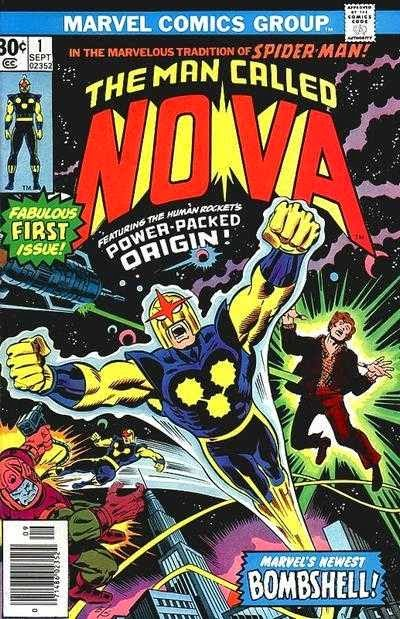And yet I digress...

My childhood is a story I already explored in the afore-linked blog post. Back to Nova and the future.

From the beginning Nova was called the Human Rocket, which is why I asked about the whereabouts of my damn rocket boots in the subtitle. Visually, the way artists draw Nova in flight looks like he has rocket boots, and since Iron Man does, this seems a logical leap.

Those of you who are REALLY comic nerds know that Nova does not have actual rocket boots. I am taking a bit of artistic license here for the sake of a clearer science object that makes more sense for my subject: the promised future that has not been delivered. In truth, Nova's power comes from the Nova-Force. The special Xandarian helmet manifests the suit, which doubles as sealed space-suit when needed, and propels Nova in flight at incredible speeds. As you can see in art from the issue, John Buscema draws Nova's rocket-like flight  as if his entire lower body is a propulsion effect, much like the characterization of Cannonball in Marvel Comics in the 1980s.

Being a human rocket seemed  like a great idea at the age of 15. The hard round, bullet-style helmet, the blasting-like rocket effect, the super-fueled joyride are all very much allusions to sex and puberty and the cosmic-powered hormones coursing through the veins of all boys at this age. To a boy in those circumstances, rocket boots seemed like the perfect fashion accessory. I was ready to explode and reach escape velocity in just a few seconds.

I am sure you "get" the allusion here.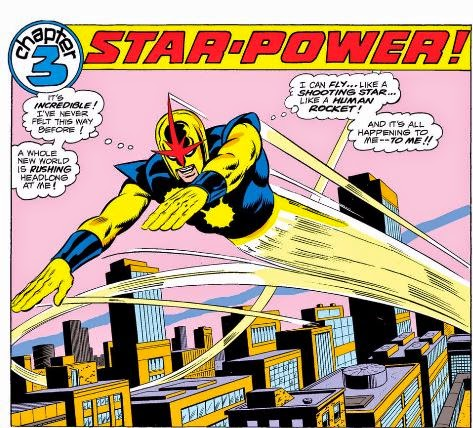I have always seen the connections between this kind of super-power and sex, but I have resisted the idea many psychologists pander (starting with Freud) that dreams of flying are always about sex.

Why aren't dreams of flying, sometimes, just about flying?

Even forty some years later, the idea of flying is very attractive just as a stress-reliever, and though this may be intermingled with desire for sex in ways that cannot be easily separated, if I think too much about Nova and his flight powers, I may dream of flying, and right now, unlike my fourteen-year-old self, the dream may be more about flying than sex.

But this blog post is not about sex; it's about Nova as the Human Rocket and the future we were promised, which has not really come to pass in some ways, and yet has exceeded our expectations in others.But first, more about NOVA.

From 1975 or so until some time in the early 1980s, I enjoyed subscriptions to some Marvel Comics that I loved, such as Uncanny X-Men (the new Claremont-Cockrum-Byrne works), Avengers, Fantastic Four, and Howard the Duck. I was also a much more frequent visitor to various news stands and had more allowance money than when I was younger, and so I was able to put together consistent and consecutive runs of many of the titles I liked beyond those I have listed, such as Iron Fist, Killraven, Legion of Superheroes,  and Daredevil. But the subscriptions ensured that I would not miss issues and that the issues would arrive in the postal mail, for the most part, in nearly pristine condition.

From 1976 to 1979, Marvel published twenty-five glorious issues of Nova -- oddly later re-named The Man Called Nova. I adored these comic books. I was a huge Nova fan from the very beginning, mainly because he was a Human Rocket. I also really liked the Spider-Men re-tread. Richard Rider (with a name just as alliterative as Peter Parker) was a teenager, like me, who is given the powers of the Nova Force -- an alien helmet and a skin-tight space suit, augmenting his teenaged body with well-sculpted muscles-- by a dying Nova Centurion, very much like the passing of the alien power ring in from the former, dying Green Lantern, to the new protector, Hal Jordan, in the Silver Age DC Comics stories.

Now, researching the origins for Nova, I am excited to learn that Marv Wolfman (who would go on to create one of my favorite comics of all time at DC in The New Teen Titans) originally conceived Nova in a fanzine in the 1960s with Len Wein. This is meaningful to me because I did my own fanzine writing in the late 1980s and early 1990s. Ten years after Wolfman's own fanzine era, working at Marvel Comics as an editor and writer, he launches Nova, which runs for a delightful twenty-five issues before flaming off. Near the end, it sputtered, going bi-monthly and with artist Carmine Infantino replacing the Buscemas, mostly Sal Buscema, who drew the majority of the issues. No quality was lost there as Infantino was highly skilled (though I prefer the Buscemas), but the look of Nova did take on that of The Flash, and Infantino's work at DC.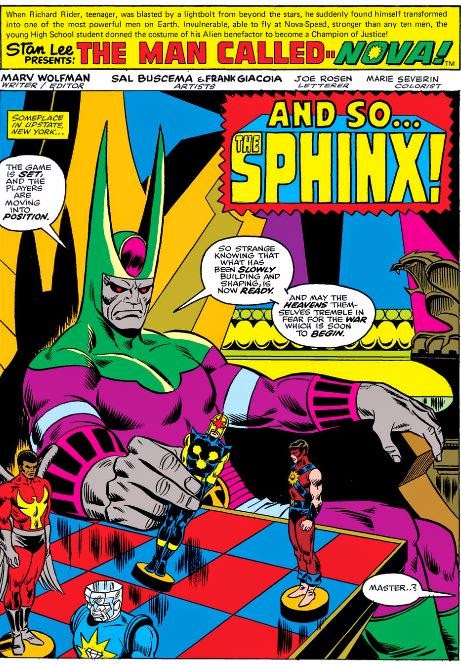Nova developed a rogue's gallery of his own villains, including Powerhouse, Condor, and Diamondhead, who later all teamed up in issue six, organized by the "Dreaded One," who we later learned was the Sphinx. The faceless Megaman terrorized Rich and his family starting in issue five and culminating in issues eight and nine, and Nova faced off against the Sphinx, finally, in issues ten and eleven. The Sphinx with his glowing eyes and over-large body might have provided the template for classic X-Men villains like Apocalypse and Mr. Sinister in the decades to follow. All the Nova villains were as brightly colored and unique as Nova himself. Also, the over-arching story that linked all the issues that first year felt very special. I had missed starting out on the ground floor with most of the comics I loved. My first issue of The Fantastic Four was #69 and my first issue of Spider-Man was #54, but here, with Nova, I was reading right from the start and I had a greater understanding of the entire scope of the story line as I had not yet missed anything.

I liked the Sphinx with his faux-Ancient-Egyptian styles and his masterminded plots, seen here in page one from issue six as he positions the players in his villainous game on a large chess board. This master planning with the chess board was very popular at the time as I recall it from an issue of Giant-Size Defenders #3 guest starring Daredevil, in which the Man Without Fear tricked the Grand Master in a game of chess.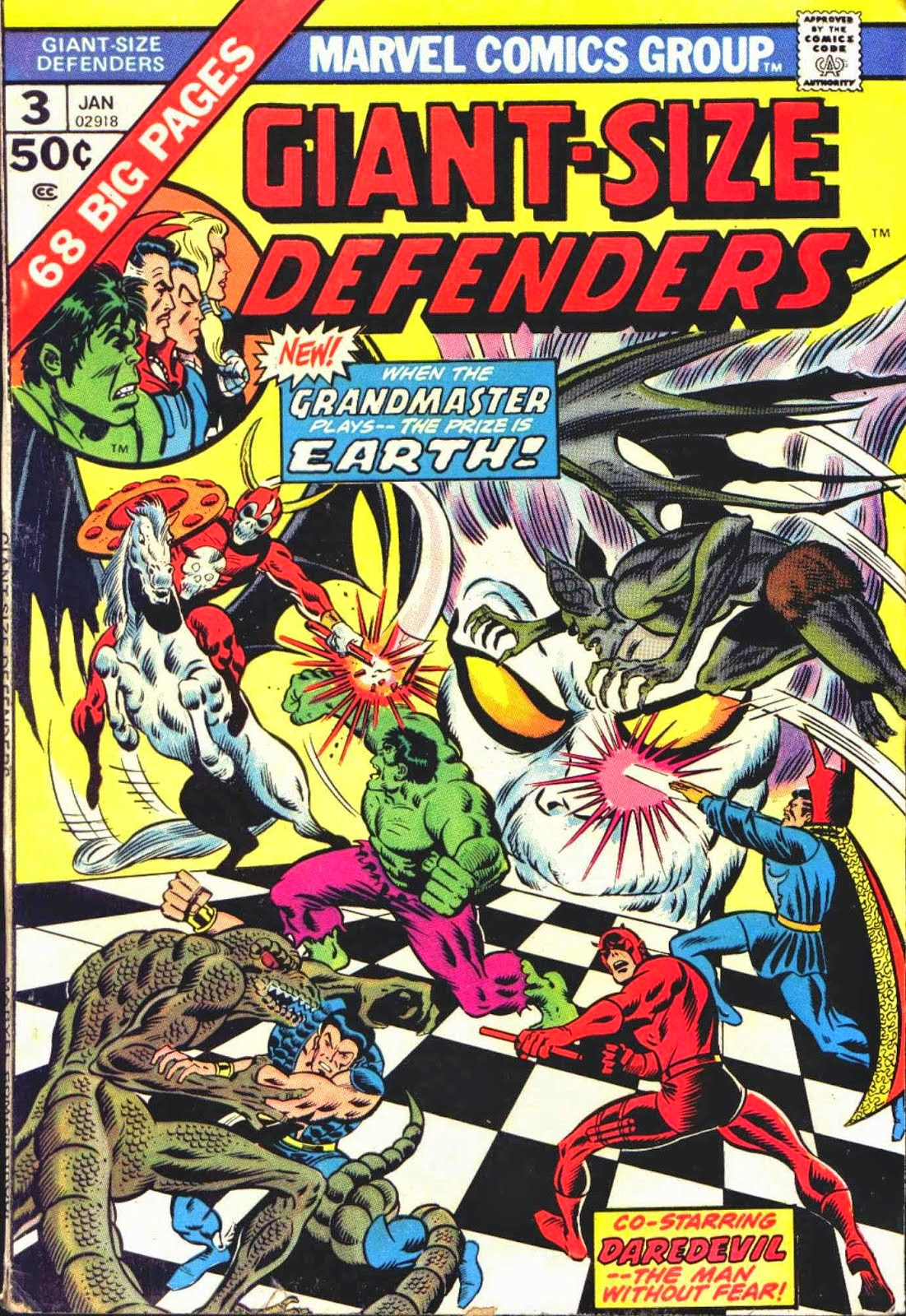Once again, I am amazed by the power of the Internet. Not only has someone written about this issue, but he has posted the entire issue online! CHECK IT OUT HERE: Giant Size Defenders #3 via Diversions of the Groovy Kind.

A brief digression. Here's two relevant pages from that comic. Follow the link if you are interested in more exploration down memory lane as Diversions of a Groovy Kind is a great blog.

To thwart the Grand Master, Daredevil engages in a simple flip of the coin game. Because of his special powers, his touch is so sensitive that he knows exactly how the disc is balanced and can flip it just right to ensure that it's heads side up in the end.

There's interesting stuff on the subject of chess in comics online. There's "Chess in Comics" by Kerry Lawless and apparently Scott McCloud's obsession with chess as a comic story HERE. For those not in the know, McCloud is famous for the seminal UNDERSTANDING COMICS book and just recently Sculptor, a graphic novel, and previously, ZOT!, a longer graphic novel that was published serially in the 1980s.

Here's those pages from the Defenders issue with the rest, again, at the following link
Giant Size Defenders #3 via Diversions of the Groovy Kind.

+=+=+=+=+=+=+=+=+=+=+=+=+=+=+=+=+=+=+=+=+=+=+=+=+=+=+=+=+=+=+=+=+=+=+=++=+=+=+=+=+=+=+=+=+=+=+=+=+=+=+=+=+=+=+=+=+=+=+=+=+=+=+=+=+=+=+=+=+=+=++=+=+=+=+=+=+=+=+=+=+=+=+=+=+=+=+=+=+=+=+=+=+=+=+=+=+=+=+=+=+=+=+=+=+=++=+=+=+=+=+=+=+=+=+=+=+=+=+=+=+=+=+=+=+=+=+=+=+=+=+=+=+=+=+=+=+=+=+=+=++=+=+=+=+=+=+=+=+=+=+=+=+=+=+=+=+=+=+=+=+=+=+=+=+=+=+=+=+=+=+=+=+=+=+=++=+=+

And now back from the digression, as I did not mean to veer off on the subject of chess in comics, though such is the beauty of my unstructured and chatty meanderings on this blog.
I had meant to use Nova as a launch point, such as it is, to write about the future as it looked from 1976 (which is now the present) and then to move the content back around to Nova again as he came up as a subject simply as one of the more interesting comic books published August 27, 2014.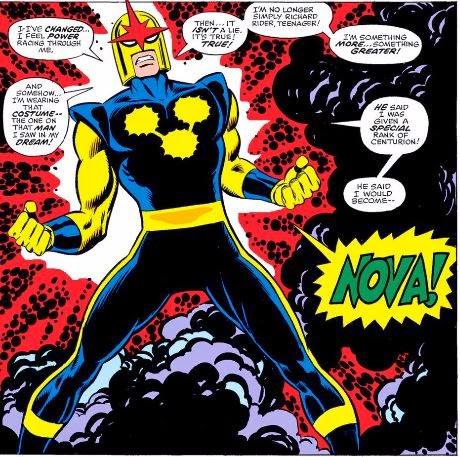I have to confess that rather than driving to where I have my comic book archive stored and spending the laborious hours needed to find my set of issues from Nova and The Man Called Nova, I simply bought the first twelve issues that had been collected in digital form. I discovered a flaw in my memory. I thought that Nova found the alien helmet, and when he donned it, the Centurion contacted him and gave him powers, much like the most recent incarnation of Nova, in which young Sam Alexander takes over the mantle of Nova when he finds his father's Centurion helmet left behind in his father's workshop. But here in Nova #1, I see Nova is blasted with an energy beam from the Centurion's ship far outside of Earth orbit, which transforms him into NOVA as shown very dramatically here in these two panels I clipped from the original comic magazine.

And so... really my analogy here sort of fails. I have little chance of being blasted by an alien's transformer energy ray that will allow me to carry on as his successor. I was thinking of rocket boots, which made me think of jet packs, which lead me into thinking about the future promised to us that has not yet materialized in many ways, and yet in some others, it has been quite unexpected.

Onward to that subject....after a few Nova resources...

Here's a but on Nova's powers.

"Powers include flight, enhanced strength, speed and durability. Nova derives his powers from an energy source called the Nova-Force, which all Nova Centurions wield. Richard Rider had the greatest potential for control as his knowledge of his power increased . Nova wears a standard Xandarian StarCorps uniform, designed to accommodate his powers without being damaged by them. In addition, the uniform has a life support function that can sustain Rider under the most extreme environmental conditions. It can act as a space suit by locking off the mouth and eyes of the helmet. The helmet can also pick up radio transmissions, as well as act as a heads-up display for tracking energy signatures." - More on Marvel.com: http://marvel.com/universe/Nova_(Richard_Rider)#ixzz3UmgowadD
NOVA LINKS
NOVA - original

Here's a cool NOVA fan page.

COMIC VINE - NOVA - ORIGINAL SERIES

Comic Vine - NOVA character page

+=+=+=+=+=+=+=+=+=+=+=+=+=+=+=+=+=+=+=+=+=+=+=+=+=+=+=+=+=+=+=+=+=+=+=++=+=+=+=+=+=+=+=+=+=+=+=+=+=+=+=+=+=+=+=+=+=+=+=+=+=+=+=+=+=+=+=+=+=+=++=+=+=+=+=+=+=+=+=+=+=+=+=+=+=+=+=+=+=+=+=+=+=+=+=+=+=+=+=+=+=+=+=+=+=++=+=+=+=+=+=+=+=+=+=+=+=+=+=+=+=+=+=+=+=+=+=+=+=+=+=+=+=+=+=+=+=+=+=+=++=+=+=+=+=+=+=+=+=+=+=+=+=+=+=+=+=+=+=+=+=+=+=+=+=+=+=+=+=+=+=+=+=+=+=++=+=+

WHERE'S MY DAMN JET PACK?

+=+=+=+=+=+=+=+=+=+=+=+=+=+=+=+=+=+=+=+=+=+=+=+=+=+=+=+=+=+=+=+=+=+=+=++=+=+=+=+=+=+=+=+=+=+=+=+=+=+=+=+=+=+=+=+=+=+=+=+=+=+=+=+=+=+=+=+=+=+=++=+=+=+=+=+=+=+=+=+=+=+=+=+=+=+=+=+=+=+=+=+=+=+=+=+=+=+=+=+=+=+=+=+=+=++=+=+=+=+=+=+=+=+=+=+=+=+=+=+=+=+=+=+=+=+=+=+=+=+=+=+=+=+=+=+=+=+=+=+=++=+=+=+=+=+=+=+=+=+=+=+=+=+=+=+=+=+=+=+=+=+=+=+=+=+=+=+=+=+=+=+=+=+=+=++=+=+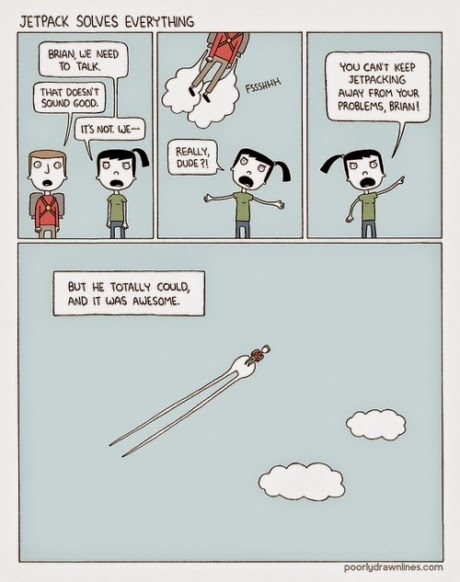JET PACKS.
ROCKET BOOTS.
FLYING CARS.

Weren't we promised that such technology would come to pass by now?

We're supposed to all have personal flight devices by now, right?

Which would you rather have a smart phone or a personal flight device?

I think I would opt for the personal flight device.

It's 2015, which looked a long way off back in 1976. I thought about this era because, after all, this is what science fiction was for. But I kind of wanted a jet pack, a flying car, a rocket sled, rocket-powered boots, or even better a personal flight device, like a flight ring, by now.

In 1976, in the aftermath of the United States Apollo program, I was convinced that 40 years onward, by 2015 at least, we would have at least one orbital space colony, a lunar colony, and even a Mars colony. I figured we would have quit our dependence on oil and coal and would have found better and cleaner means of producing energy. And we have, but we have not replaced our usage yet.
I felt assured by the SF writing world that new technology would be developed to get us to the next star system by 2015, Alpha Centauri being likely as its the closest star system with the closest earth-like planet. And if our near light travel to this system did not cause a time traveling anomaly thrusting our astronauts into a future in which apes have taken over the Planet Earth, then we might make contact with an alien species. Though cool and mysterious, I never really "bought" the contact stories with an ineffable obelisk, a la 2001 a Space Odyssey, or certainly a few years later, the cryptic yet helpful aliens who create a whole new planetary system by transforming Jupiter into a star, as in 2010 - The year we make contact.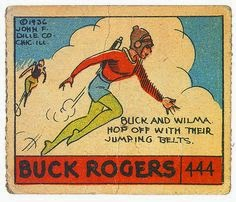Amid all this amazing space travel and communion with alien species, we would all have flying cars and/or our own personal flight devices. Sure, some kind of jet or rocket pack or boot rockets would be cool, but much more elegant would be the Legion of Superheroes flight rings with their force field space suits.

Computers would remain large machines with banks of circuitry, cube or large panel screens, and discs for storage. I knew there would be some computer communications, but barely conceived of the Internet as a young boy in the mid-1970s, let alone the portable computing power that I would enjoy now forty years in the future. Star Trek showed computers as large devices and storage on disks. Though some portable devices existed they were like giant clip boards and not at all like my compact smart phone or touch screen tablet.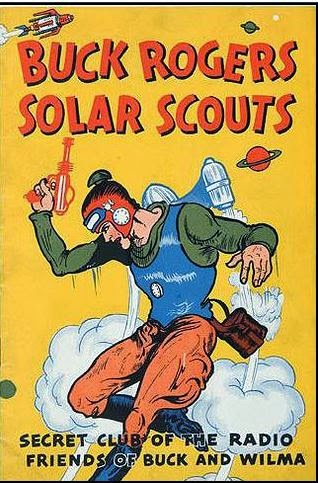And so, we have future promised and not delivered and also a future not promised that has been delivered beyond wildest expectations. We do not have personal flight devices, but we have smart phones.

Apparently, I am not the only one who has thought about this issue. There's a great deal of activity online for "where is my damn jet pack?" I searched jet pack as I figured it may have better results than "rocket boots." Most searches about rocket boots provide results for various games or Iron Man.

Check out these delightful links, especially the Ellis stuff.

+=+=+=+=+=+=+=+=+=+=+=+=+=+=+=+=+=+=+=+=+=+=+=+=+=+=+=+=+=+=+=+=+=+=+=++

When Do I get my jet pack?

Where is my damn jet pack?

Your flying cars are on their way!

First jet pack?

It's 2007 and where's my DAMN JET PACK?

Jet Pack Solves Everything - source

ELLIS - Someone stole your future - 2007

ELLIS - Rachel Armstrong on Where Our Future Went -2013

ELLIS - Future Underground - 2004

ELLIS via SEQUART Doktor Sleepless - Unfinished Apocalypse

COMIC BOOK PHILOSOPHER - Ellis - Doktor Sleepless - the Future

WARREN ELLIS answers your questions about THE FUTURE

sidenote - ELLIS - The Final Solution - evil - dogs

+=+=+=+=+=+=+=+=+=+=+=+=+=+=+=+=+=+=+=+=+=+=+=+=+=+=+=+=+=+=+=+=+=+=+=++

+=+=+=+=+=+=+=+=+=+=+=+=+=+=+=+=+=+=+=+=+=+=+=+=+=+=+=+=+=+=+=+=+=+=+=++=+=+=+=+=+=+=+=+=+=+=+=+=+=+=+=+=+=+=+=+=+=+=+=+=+=+=+=+=+=+=+=+=+=+=++=+=+=+=+=+=+=+=+=+=+=+=+=+=+=+=+=+=+=+=+=+=+=+=+=+=+=+=+=+=+=+=+=+=+=++=+=+=+=+=+=+=+=+=+=+=+=+=+=+=+=+=+=+=+=+=+=+=+=+=+=+=+=+=+=+=+=+=+=+=++=+=+=+=+=+=+=+=+=+=+=+=+=+=+=+=+=+=+=+=+=+=+=+=+=+=+=+=+=+=+=+=+=+=+=++=+=+
SPOILERS SPOILERS SPOILERS SPOILERS SPOILERS
SPOILERS SPOILERS SPOILERS SPOILERS SPOILERS
SPOILERS SPOILERS SPOILERS SPOILERS SPOILERS
SPOILERS SPOILERS SPOILERS SPOILERS SPOILERS
RE: SPOILERS: I don't have to explain that you read at your own risk, right?
=+=+=+=+=+=+=+=+=+=+=+=+=+=+=+=+=+=+=+=+=+=+=+=+=+=+=+=+=+=+=+=+=+=++=+=+=+=+=+=+=+=+=+=+=+=+=+=+=+=+=+=+=+=+=+=+=+=+=+=+=+=+=+=+=+=+=+=+=++=+=+

CBR FOR AUGUST 13 2014

IGN FOR AUGUST 13 2014

WEEKLY COMICS LIST

Since I devoted so much content to Nova and the promise of flight in our future tech, I am limiting myself to general discussion of ordering of these comics and just exploration of one individual issue, Guardians of the Galaxy issue #18.

Surely, Guardians of the Galaxy issue #18 hits the top of the stack this week primarily because of Nova on the cover and answers to what happened to Richard Rider. It helped that Ed McGuiness joined Bendis on this issue as I think McGuiness is a solid artist.

As for the other ranks, Guardians of the Galaxy issue #18 narrowly beats these comics, which all could have earned the number one slot: Aquaman #34, Superman #34, Fantastic Four #009, and
Savage Hulk #003. Aquaman and Fantastic Fourare always going to hit the top of my stack. Superman had been ranked high recently due to the arrival of John Romita, Jr. but by August, two months later, I had soured on that somewhat and the book fell to third. With Alan Davis on art and the retro story of the early X-Men, Savage Hulk ranked well this week as did Amazing Spider-Man: Learning to Crawl #01.4, which I enjoyed immensely. Saga #22 and Outcast #3 could be top ranked books as well and only fall lower because I feel that they take more brain power to read, and I want to save them until I have consumed several faster reads, which is the ONLY reason a book like The New 52 - Future's End #17 would be ranked ahead of them.

More recently, I have been ranking Batman Eternal higher. This book just hit near the top of my recent list as I am typing this in April 2015, and the title just hit issue #52, which is its conclusion.

As of this writing, Silver Surfer and Uncanny Avengers are still back logged, and I have stopped buying Batman/Superman.

Recently, I have moved up Avengers and its companion titles, even though I continually frustrated with Hickman's sprawling and confusing story. Though I have read the issues listed here, several more recent issues of All New Invaders and The Flash are in my current back log.

That's all for now. This one was a LONG time in the making... Moving now to monthly entries. That will be difficult enough! :-)

RANKED COMICS from AUGUST 27, 2014

Guardians of the Galaxy - Original Sin #18
Aquaman #34
Superman #34
Fantastic Four #009
Savage Hulk #003
All New X-Men #031
Amazing Spider-Man: Learning to Crawl #01.4
The New 52 - Future's End #17
Saga #22
Outcast #3
Avengers #34
All New Invaders #009
Batman Eternal #21
The Flash #34

BACKLOG
Batman/Superman #13
Silver Surfer #005
Uncanny Avengers #023
+=+=+=+=+=+=+=+=+=+=+=+=+=+=+=+=+=+=+=+=+=+=+=+=+=+=+=+=+=+=+=+=+=+=+=++=+=+=+=+=+=+=+=+=+=+=+=+=+=+=+=+=+=+=+=+=+=+=+=+=+=+=+=+=+=+=+=+=+=+=++=+=+=+=+=+=+=+=+=+=+=+=+=+=+=+=+=+=+=+=+=+=+=+=+=+=+=+=+=+=+=+=+=+=+=++=+=+=+=+=+=+=+=+=+=+=+=+=+=+=+=+=+=+=+=+=+=+=+=+=+=+=+=+=+=+=+=+=+=+=++=+=+=+=+=+=+=+=+=+=+=+=+=+=+=+=+=+=+=+=+=+=+=+=+=+=+=+=+=+=+=+=+=+=+=++=+=+

THE ORIGINAL RUN OF NOVA comics COVER GALLERY

Okay, not too much over art directly on the blog. I pre-loaded this stuff and put the link here. Enjoy.

NOVA COVER GALLERY

+=+=+=+=+=+=+=+=+=+=+=+=+=+=+=+=+=+=+=+=+=+=+=+=+=+=+=+=+=+=+=+=+=+=+=++=+=+=+=+=+=+=+=+=+=+=+=+=+=+=+=+=+=+=+=+=+=+=+=+=+=+=+=+=+=+=+=+=+=+=++=+=+=+=+=+=+=+=+=+=+=+=+=+=+=+=+=+=+=+=+=+=+=+=+=+=+=+=+=+=+=+=+=+=+=++=+=+=+=+=+=+=+=+=+=+=+=+=+=+=+=+=+=+=+=+=+=+=+=+=+=+=+=+=+=+=+=+=+=+=++=+=+=+=+=+=+=+=+=+=+=+=+=+=+=+=+=+=+=+=+=+=+=+=+=+=+=+=+=+=+=+=+=+=+=++=+=+

### Nova and the Man Called Nova Cover Gallery

I was going to put this in my entry on Comics from 1408.27, but then I decided to move it into its own blog entry with page break. So here you go...